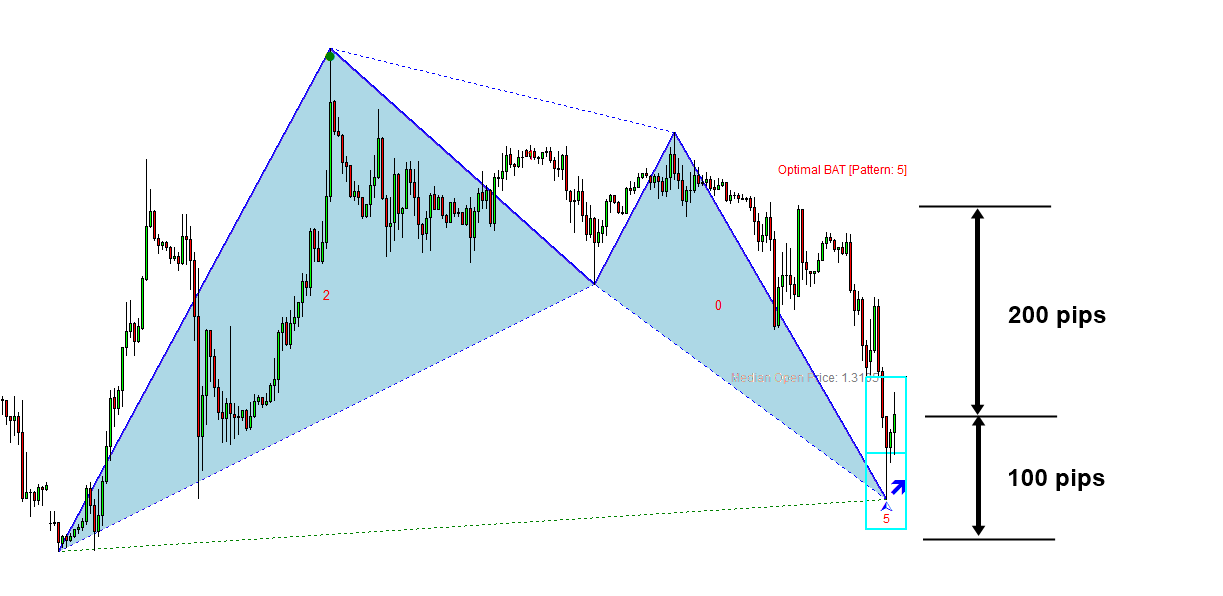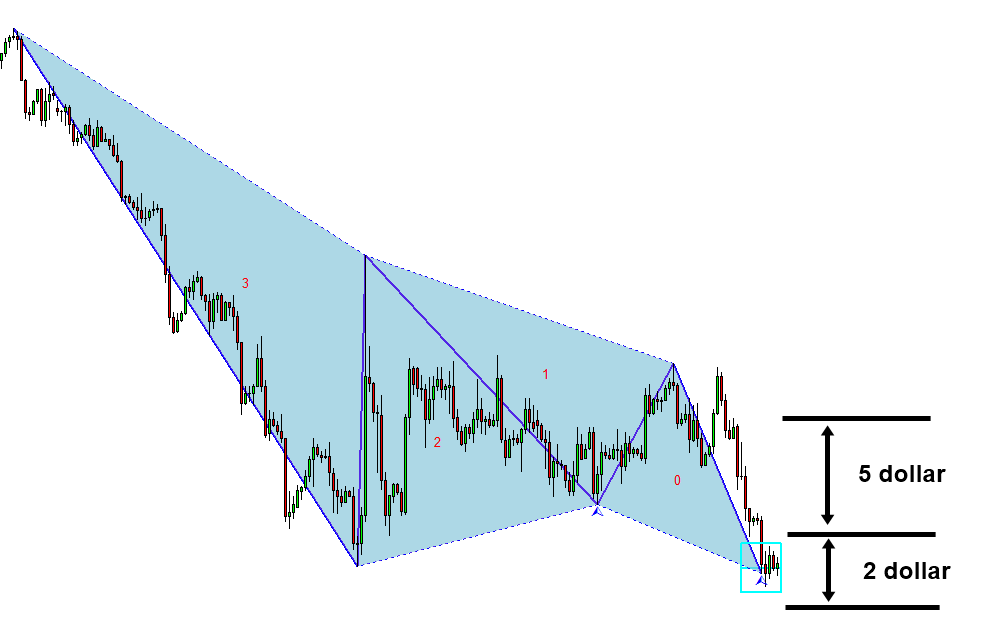Questions on Risk Management with Profitable Patterns

.

1. Currently, Apple’s stock price is 200 dollar. You have an account size of 50,000 dollar. You are trading with 1% risk. Calculate number of share to buy if your stop loss is at 180 dollar.

.

2. Currently, Google’s stock price is 1000 dollar. You have an account size of 50,000 dollar. You are trading with 1% risk. Calculate number of share to buy if your stop loss is at 950 dollar.

.

3. Currently, EURUSD is at 1.2000. You have an account size of 50,000 dollar. You are trading with 1% risk. Calculate position size if your stop loss is taking 30 pips and pip value is 10 dollar per 1 lot contract.

.

4. Currently, GBPUSD is at 1.2500. You have an account size of 50,000 dollar. You are trading with 1% risk. Calculate position size if your stop loss is taking 50 pips and pip value is 10 dollar per 1 lot contract.

.

5. You have made three trades with stop loss size 100 dollar and take profit size 150 dollar. You had two winning trades and one losing trade. Calculate your profit and loss if your account size is 20,000 us dollar.

.

6. You have made five trades with stop loss size 200 dollar and take profit size 500 dollar. You had two winning trades and three losing trade. Calculate your profit and loss if your account size is 30,000 us dollar.

7. You have made ten trades with stop loss size 200 dollar and take profit size 600 dollar. You had four winning trades and six losing trade. Calculate your profit and loss if your account size is 50,000 us dollar.

.

8. You have made ten trades with stop loss size 200 dollar and take profit size 600 dollar. You success rate is 0.4. Calculate your profit and loss if your account size is 50,000 us dollar. Use hypothetical profit formula for the calculation.

.

9. You have made ten trades with stop loss size 200 dollar and take profit size 600 dollar. You had 35 winning trades and 65 losing trade. Calculate your profit and loss if your account size is 50,000 us dollar.

.

10. You have made ten trades with stop loss size 200 dollar and take profit size 600 dollar. You success rate is 0.35. Calculate your profit and loss if your account size is 50,000 us dollar. Use hypothetical profit formula for the calculation.

.

11. You success rate is 0.1 and Reward/Risk ratio = 10. Calculate hypothetical profit per trade if your account size is 50,000 us dollar and your risk is 1%.

.

12. You success rate is 0.9 and Reward/Risk ratio = 0.1. Calculate hypothetical profit per trade if your account size is 50,000 us dollar and your risk is 1%.

.

13. Calculate breakeven win rate if your stop loss size is 100 dollar and your take profit size is 300 dollar.

.
14. Calculate breakeven win rate if your stop loss size is 200 dollar and your take profit size is 300 dollar.

.
15. Calculate breakeven win rate if your stop loss size is 300 dollar and your take profit size is 30 dollar.

.
16. Among these four trading setup, which one requires highest probability to win?
a) Trade with AB=CD pattern with stop loss size 20 dollar and take profit size 50 dollar
b) Trade with Elliott Wave 12345 Pattern with stop loss size 200 dollar and take profit size 500 dollar
c) Trade with Butterfly Pattern with stop loss size 5 dollar and take profit size 3 dollar
d) Trade with Eagle pattern with stop loss size 1000 dollar and take profit size 3000 dollar

.
17. Among these four trading setup, which one requires lowest probability to win?
a) Trade with AB=CD pattern with stop loss size 20 dollar and take profit size 50 dollar
b) Trade with Elliott Wave 12345 Pattern with stop loss size 200 dollar and take profit size 500 dollar
c) Trade with Butterfly Pattern with stop loss size 5 dollar and take profit size 3 dollar
d) Trade with Eagle pattern with stop loss size 1000 dollar and take profit size 3000 dollar

.

18. You have an account size 50,000 dollar and you want to achieve 20,000 dollar profit (i.e. 40% profit). Say you can achieve 35% success rate for Reward/Risk ratio = 3. How many trades do you need to achieve 20,000 dollar profit (i.e. grow your account to 70,000 dollar). Say you are using 1% account risk per trade.

.
19. You have an account size 10,000 dollar and you want to achieve 10,000 dollar profit (i.e. 100% profit). Say you can achieve 38% success rate for Reward/Risk ratio = 3. How many trades do you need to achieve 10,000 dollar profit (i.e. grow your account to 20,000 dollar). Say you are using 1% account risk per trade.

.
20. You have an account size 100,000 dollar and you want to achieve 50,000 dollar profit (i.e. 50% profit). Say you can achieve 32% success rate for Reward/Risk ratio = 3. How many trades do you need to achieve 50,000 dollar profit (i.e. grow your account to 150,000 dollar). Say you are using 1% account risk per trade.

.
21. Calculate your position size for following EURUSD trade with account size 20,000 dollar and 2% risk (Stop loss size = 100 pips and take profit size = 200 pips). Also calculate breakeven success rate..

22. Calculate number of shares for following trade with account size 20,000 dollar and 2% risk (Stop loss size = 2 dollars and take profit size = 5 dollars). Also calculate breakeven success rate..
23. Describe meaning of success rate

.

24. Describe meaning of breakeven win rate

.

25. Give some thought about the advantage of using Reward/Risk ratio over Risk/Reward ratio

Below is the landing page for Harmonic Pattern Plus, Harmonic Pattern Scenario Planner and X3 Chart Pattern Scanner in MetaTrader. All these products are also available from www.mql5.com too.